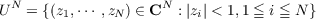#### Vol. 29, No. 3, 1969

 Download this articleFor screen For printingRecent Issues Vol. 325: 1 Vol. 324: 1  2 Vol. 323: 1  2 Vol. 322: 1  2 Vol. 321: 1  2 Vol. 320: 1  2 Vol. 319: 1  2 Vol. 318: 1  2Online Archive Volume: Issue:The Journal Subscriptions Editorial Board Officers Contacts Submission Guidelines Submission Form Policies for Authors ISSN: 1945-5844 (e-only) ISSN: 0030-8730 (print) Special Issues Author Index To Appear Other MSP Journals
Extending bounded holomorphic functions from certain subvarieties of a polydisc

### Herbert James Alexander

Vol. 29 (1969), No. 3, 485–490
##### Abstract

Let E be a subvariety of the unit polydiscsuch that E is the zero set of a holomorphic function f on UN, i.e., E = Z(f) where Z(f) = {z UN : f(z) = 0}. This amounts to saying that E is a subvariety of pure dimension N 1. In  Walter Rudin proved that if E is bounded away from the torus TN = {(z1,,zN) CN : |zi| = 1,1 i N}, then there is a bounded holomorphic function F on UN such that E = Z(F). Call such a subvariety E, that is, a pure N 1 dimensional subvariety of UN bounded from TN, a Rudin variety. We are interested in the following question: When is it possible to extend every bounded holomorphic function on a Rudin variety E to one on UN? Examples show this is not always possible. We will say that a pure N 1 dimensional subvariety E of UN is a special Rudin variety if there exists an annular domain QN = {(z1,,zN) CN : r < |zi| < 1,1 i N} for some r(0 < r < 1) and a δ > 0 such that

(i) E QN = and

(ii) if 1 k N and (z,α,z′′) (Qk1 × U × QNk) E and (z,β,z′′) (Qk1 ×U ×QNk) E and αβ, then |αβ|δ. Obviously (i) implies that a special Rudin variety is a Rudin variety. We have the

Theorem. If E is a special Rudin variety in UN, then there exists a bounded linear transformation T : H(E) H(UN) (where H is the corresponding Banach space of bounded holomorphic functions under sup norm) which extends each bounded holomorphic function on E to one on UN.

Primary: 32.20
##### Milestones